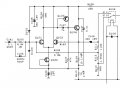# Discrete FET differential amp voltage follower with positive feedback.

#### brianmk

Joined Dec 23, 2016
21
Below is a section of the schematic of the front end of a Kenwood VT-181 AC Millivoltmeter.
It's a fairly conventional discrete FET differential amp acting as a voltage follower / impedance converter.
The output feeds a 0dB / 30dB attenuator selected by a relay.

I'm puzzled about the positive feedback path via R106.
As well as providing the small input current required by Q101 (the input is AC coupled) it appears to slightly lift the hf frequency response in conjunction with R103 and C104.
What I don't understand is that the hf lift will surely depend upon the impedance of whatever is connected to the input.
If it's connected to a high impedance source then it won't have much effect.
Am I missing something?#### crutschow

Joined Mar 14, 2008
27,908
R106 provides negative (not positive) feedback to stabilize the circuit DC bias point.

The input impedance is basically determined by the value of R106 and the stray FET and circuit capacitance.
So the source impedance needed for a good measurement depends upon the frequency being measured due to the stray capacitance load, which is probably in the neighborhood of 50pF.

#### brianmk

Joined Dec 23, 2016
21
R106 provides negative (not positive) feedback to stabilize the circuit DC bias point.
Sorry but I still don't understand. R106 is not AC decoupled, it's connected directly to the signal output. Surely that means it will provide a positive signal feedback path?

The input impedance is basically determined by the value of R106 and the stray FET and circuit capacitance.
So the source impedance needed for a good measurement depends upon the frequency being measured due to the stray capacitance load, which is probably in the neighborhood of 50pF.
Yes, I realise that. The point I was trying to make is that the effect of R103 & C104 in lifting the hf response will surely vary depending on what's connected to the input.
If I connect the input to a voltage source with 1M impedance, then R103 & C104 will have little effect compared with connecting to a voltage source with say 1K impedance.
An LTSpice simulation confirms this.

Last edited:

#### brianmk

Joined Dec 23, 2016
21
BTW There can be no negative DC feedback via R106 because of C108, so it's not there to stabilise the DC operating point.
As far as the DC operating point is concerned, R106 is connected to ground via R112 and R113.

Last edited:

#### brianmk

Joined Dec 23, 2016
21
I think I've now figured this out...

R106 is bootstrapped to increase the amp's input resistance.
If R106 were simply connected to ground, the amp's resistive input impedance would be around 3.3M.
However, since the other side of R106 is connected to the amp's output (which has a voltage gain of approximately one), the signal voltage across the resistor (and the current through it) is virtually zero, so the input resistance appears much higher (tens of Megohms).

I think R103 and C104 compensate for the gate capacitance of Q101, the capacitance of the two reverse biased protection diodes along with other stray capacitance.

#### OBW0549

Joined Mar 2, 2015
3,566
I think I've now figured this out...

R106 is bootstrapped to increase the amp's input resistance.
If R106 were simply connected to ground, the amp's resistive input impedance would be around 3.3M.
However, since the other side of R106 is connected to the amp's output (which has a voltage gain of approximately one), the signal voltage across the resistor (and the current through it) is virtually zero, so the input resistance appears much higher (tens of Megohms).
That's it, exactly. R106 and R111-R113 comprise a bootstrapped bias arrangement for Q101 which raises the effective input impedance to around 52 MΩ for the given resistor values.

#### crutschow

Joined Mar 14, 2008
27,908
There can be no negative DC feedback via R106 because of C108, so it's not there to stabilise the DC operating point.
Yes, I missed that in my cursory look at the circuit, also the signal inversion caused by Q103.Must have stayed up too late.